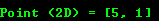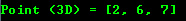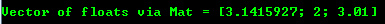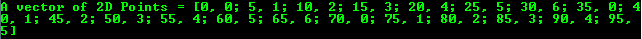OpenCV Mat —— 基本的图像容器

1
0
01. 云栖社区>
2. 博客>
3. 正文

OpenCV Mat —— 基本的图像容器

目标

Mat

OpenCV 项目大约在 2001 年推出，之前主要是提供了 C 接口并通过名为 IplImage  的 C 结构体来处理内存中的图像。在一些老的教程和学习材料中你经常会看到这个结构体。使用这个结构体的问题是让 OpenCV 严重受限于 C 语言的特性和缺点，最大的问题就是需要进行手工内存管理。它要求用户必须小心的操作内存的分配和释放。对一些小程序而言，这不是什么大问题，但是一旦你的代码量增长越来越迅速时，这个问题变得非常严重。

Mat 是一个类，包含了阵列的头（阵列大小、存储的方法以及存储地址等等）和指向阵列点阵数据的指针（维度取决于存储的方法）。阵列的头部大小是一个常量，不同图片的头部存放的阵列大小是不同的。

OpenCV 是一个图像处理库。其包含大量各种图像处理函数。为了满足计算的要求，绝大多数时间你都会使用多个 OpenCV 函数。例如传递图片给某个函数是经常需要做的。我们别忘了我们正在讨论图像处理算法，这往往是非常沉重的计算。最后我们需要做的是进一步提升程序的速度，减少潜在的不必要的大图片拷贝。

Mat A, C;  // 创建头部

Mat B(A);  // 使用拷贝构造函数

C = A;     // 赋值操作

Mat D (A, Rect(10, 10, 100, 100) ); // 使用矩形
Mat E = A(Range::all(), Range(1,3)); // 使用行列边界

Mat F = A.clone();
Mat G;
A.copyTo(G);

• 为 OpenCV 函数输出图像的内存分配是自动的（除非特别说明）
• 使用 OpenCV 的 C++ 接口不需要考虑内存管理的问题
• 赋值操作和拷贝构造函数只拷贝了头部信息
• 图像底层的矩阵可以通过 clone() 和 copyTo() 函数进行拷贝

存储函数

• RGB 最常用，因为跟我们的眼睛识别方式类似，但需要注意的是 OpenCV 显示系统用的是 BGR 色彩
• HSV 和 HLS 将颜色分解成色调、饱和度和亮度组件，用来描述色彩更为直观。它可以让你忽略值组件，使你的算法对输入图像的光照条件不那么敏感
• YCrCb 常用语 JPEG 图像格式
• CIE L*a*b 是一个感知均匀的色彩空间，可以方便的用来计算从一个颜色到另外一个颜色的差异。

显式的创建 Mat 对象

• Mat() 构造函数

Mat M(2,2, CV_8UC3, Scalar(0,0,255));
cout << "M = " << endl << " " << M << endl << endl;

CV_[The number of bits per item][Signed or Unsigned][Type Prefix]C[The channel number]

• 使用 C/C++ 数组并通过构造函数初始化

int sz = {2,2,2};
Mat L(3,sz, CV_8UC(1), Scalar::all(0));

上述示例显示如何创建一个超过 2 个维度的阵列。指定阵列的维度数，并传递包含每个维度大小的指针。

• 为已有的 IplImage 指针创建一个头部：

IplImage* img = cvLoadImage("greatwave.png", 1);
Mat mtx(img); // convert IplImage* -> Mat

• Create() 函数:

M.create(4,4, CV_8UC(2));
cout << "M = "<< endl << " "  << M << endl << endl;

• MATLAB 风格的初始化: zeros()ones()eye(). 指定大小和数据类型:

Mat E = Mat::eye(4, 4, CV_64F);
cout << "E = " << endl << " " << E << endl << endl;

Mat O = Mat::ones(2, 2, CV_32F);
cout << "O = " << endl << " " << O << endl << endl;

Mat Z = Mat::zeros(3,3, CV_8UC1);
cout << "Z = " << endl << " " << Z << endl << endl;

• 对于一些小的阵列你可以使用逗号隔开初始化方法:

Mat C = (Mat_<double>(3,3) << 0, -1, 0, -1, 5, -1, 0, -1, 0);
cout << "C = " << endl << " " << C << endl << endl;

• 为一个已有的 Mat 对象创建一个新的头部，并进行 clone() 后者 copyTo() .

Mat RowClone = C.row(1).clone();
cout << "RowClone = " << endl << " " << RowClone << endl << endl;Mat R = Mat(3, 2, CV_8UC3);
randu(R, Scalar::all(0), Scalar::all(255));

输出格式化

• 默认

cout << "R (default) = " << endl <<        R           << endl << endl;• Python

cout << "R (python)  = " << endl << format(R,"python") << endl << endl;• 逗号分隔的值 (CSV)

cout << "R (csv)     = " << endl << format(R,"csv"   ) << endl << endl;• Numpy

cout << "R (numpy)   = " << endl << format(R,"numpy" ) << endl << endl;• C

cout << "R (c)       = " << endl << format(R,"C"     ) << endl << endl;其他常用条目的输出

• 2D Point

Point2f P(5, 1);
cout << "Point (2D) = " << P << endl << endl;• 3D Point

Point3f P3f(2, 6, 7);
cout << "Point (3D) = " << P3f << endl << endl;• std::vector via cv::Mat

vector<float> v;
v.push_back( (float)CV_PI);   v.push_back(2);    v.push_back(3.01f);

cout << "Vector of floats via Mat = " << Mat(v) << endl << endl;• std::vector of points

vector<Point2f> vPoints(20);
for (size_t i = 0; i < vPoints.size(); ++i)
vPoints[i] = Point2f((float)(i * 5), (float)(i % 7));

cout << "A vector of 2D Points = " << vPoints << endl << endl;+ 关注